# General Description of the Python Processors

The Python Processors give users the ability to add Python codes to a ONE DATA Workflow. These are the types of Python Processors available:

# Example Scripts

## Simple Operations in Python

```output = []
for x in range(1, @fizz@+1):
#print(x)
insert = ''
if x%3 == 0:
insert = 'fizz'
if x%5 == 0:
insert = insert + 'buzz'
if x%3!=0 and x%5!=0:
insert = str(x)

output.append([insert])
print(output)

### Processor: Python Script Data Generator

Requirement: ONE DATA variable of type Integer and name “fizz”

Procedure:

• The Python code replaces the row counter with "fizz", "buzz" or "fizzbuzz" in specific cases
• If the row number of the Int – Variable can be divided by 3 with a remainder of 0, the code replaces the counter with "fizz"
• If the row number of the Int – Variable can be divided by 5 with a remainder of 0, the code replaces the counter with "buzz"
• If the row number of the Int – Variable can be divided by 3 and 5 with a remainder of 0, the code replaces the counter with "fizzbuzz"

Output:

In this example the results are stored within a list. As the Python Processor must return a data table, the results are transformed before being returned to ONE DATA.

For this operation the function "od_output.add_data()" is used.

## Create a Bar Chart With Conditional Coloring

```from onelogic.odpf import ImageType
import pandas as pd
import numpy as np
import matplotlib.pyplot as plt

# write a complete new dataset and create a plot of it
index = range(10)
df = pd.DataFrame(np.random.randn(10,1), index=index, columns=list('A'))

# color negative values red, positive green
colors = []
for index, row in df.iterrows():
if row['A'] < 0:
colors.append('r')
else:
colors.append('g')

fig = plt.figure()
ax.bar(x=df.index, height=df['A'], color=colors)

Processor: Python Script Data Generator

Procedure:

• Generating a new dataset with the length of rows = 10. A vector of 10 random numbers following a normal distribution are generated and stored in column "A"
• The colors are set according to the shape of the 10 numbers (whether they are < 0 or >= 0)
• A bar chart is created and colors are set accordingly

Output:

• Creates a bar chart of 10 random values
• Bars for values < 0 are colored red
• Bars for values => 0 are colored green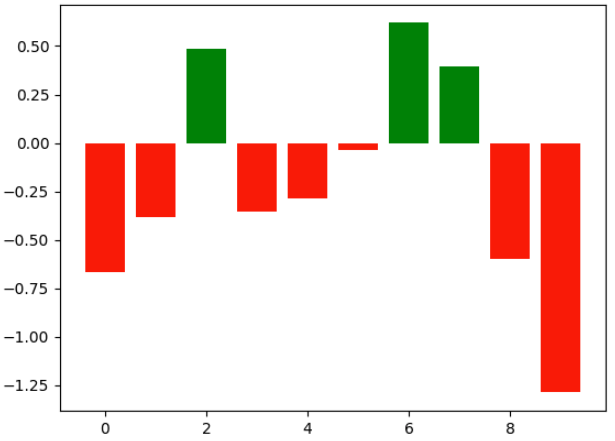## Basic Work With Iris Dataset

```from onelogic.odpf import ImageType
import numpy as np
from pandas import *
import matplotlib.pyplot as plt

dataset = od_input['input'].get_as_pandas()

# create datasets for the different varieties
setosa = dataset[dataset['variety']=='Setosa']
versicolor = dataset[dataset['variety']=='Versicolor']
virginica = dataset[dataset['variety']=='Virginica']

# get summary statistics of the data
print(setosa.describe())
print(versicolor.describe())
print(virginica.describe())

# create figure/plot
plt.figure()
fig,ax=plt.subplots(1,2,figsize=(21, 10))

# plot the different varieties depending on sepal and petal values
setosa.plot(x="sepal_length", y="sepal_width", kind="scatter",ax=ax,label='setosa',color='r')
versicolor.plot(x="sepal_length",y="sepal_width",kind="scatter",ax=ax,label='versicolor',color='b')
virginica.plot(x="sepal_length", y="sepal_width", kind="scatter", ax=ax, label='virginica', color='g')

setosa.plot(x="petal_length", y="petal_width", kind="scatter",ax=ax,label='setosa',color='r')
versicolor.plot(x="petal_length",y="petal_width",kind="scatter",ax=ax,label='versicolor',color='b')
virginica.plot(x="petal_length", y="petal_width", kind="scatter", ax=ax, label='virginica', color='g')

ax.set(title='Sepal comparasion ', ylabel='sepal-width')
ax.set(title='Petal Comparasion',  ylabel='petal-width')
ax.legend()
ax.legend()

Processor: Python-Script Single Input

Requirement: The Iris dataset (attached at the end of the article) and a Workflow that loads it (e.g. with a Data Table Load Processor) and passes it as input to the Python Processor.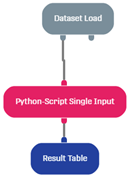Procedure:

• Creating three datasets with 50 observations each for the three species of Iris flowers (Setosa, Versicolor, Virginica)
• Generating the summary statistics of each species for the sepal length / width and petal length / width
• Plotting the three species datasets in a scatterplot with either x = ‘sepal_length’, y = ‘sepal_width’ or x = ‘petal_length’, y = ‘petal_width’
• Adding labels to the plots ("Sepal Comparison", "Petal Comparison")

Output:

• Lines 13-15 produce summary statistics in the log of the Python Processor: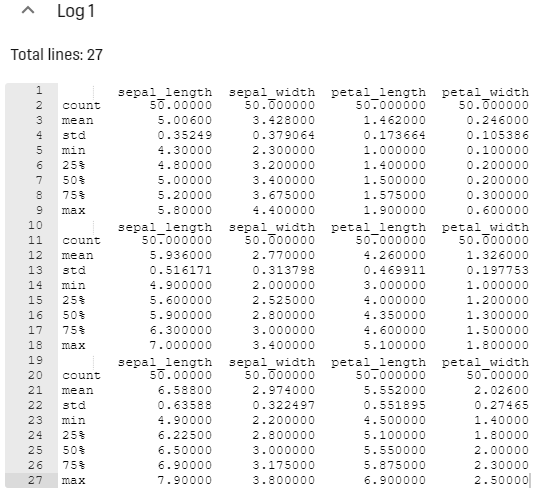• Scatter plots of the three species' sepal and petal: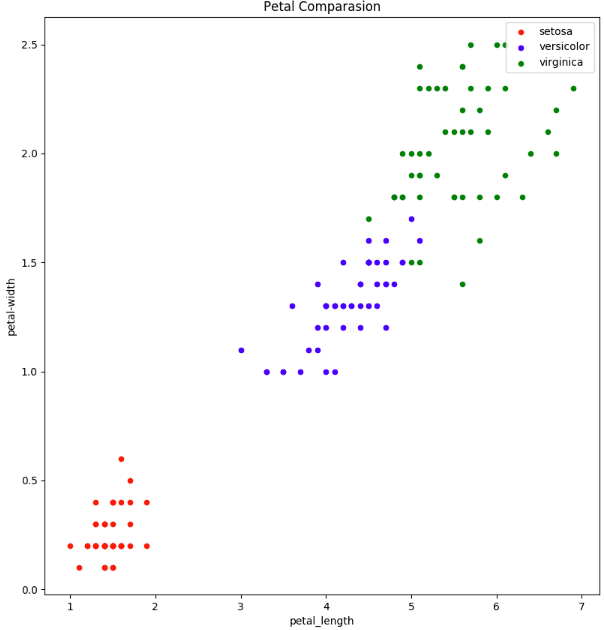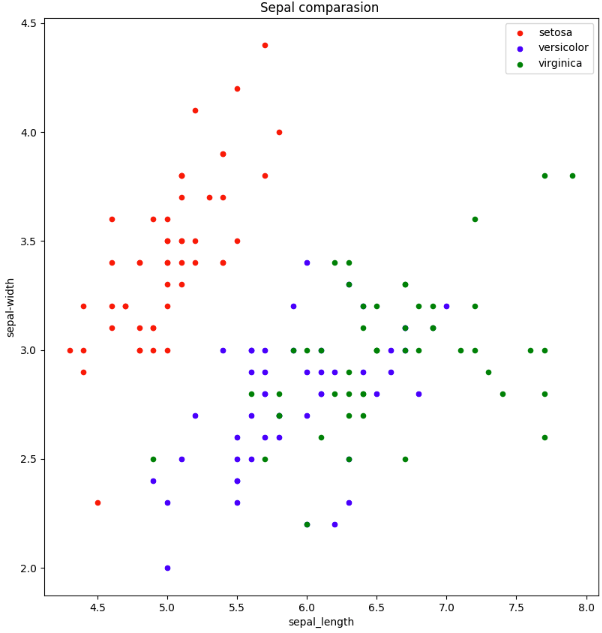• Result Table with summary statistics for all observations: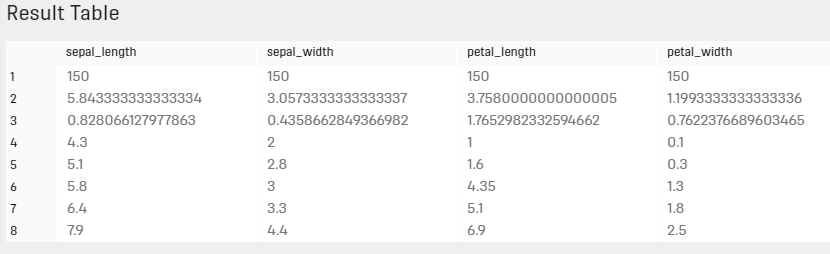## Machine Learning on Iris Dataset With sklearn & pandas

```from onelogic.odpf import ImageType
import pandas as pd
from pandas.plotting import scatter_matrix
import matplotlib.pyplot as plt
from sklearn import model_selection
from sklearn.metrics import classification_report
from sklearn.metrics import confusion_matrix
from sklearn.metrics import accuracy_score
from sklearn.linear_model import LogisticRegression
from sklearn.tree import DecisionTreeClassifier
from sklearn.neighbors import KNeighborsClassifier
from sklearn.discriminant_analysis import LinearDiscriminantAnalysis
from sklearn.naive_bayes import GaussianNB
from sklearn.svm import SVC

dataset = od_input['input'].get_as_pandas()

# splitting in training and validation sets
array = dataset.values
X = array[:,0:4]
Y = array[:,4]
validation_size = 0.20
seed = 7
X_train, X_validation, Y_train, Y_validation = model_selection.train_test_split(X, Y, test_size=validation_size, random_state=seed)

# defining the Models that should be used
models = []
models.append(('LR', LogisticRegression()))
models.append(('LDA', LinearDiscriminantAnalysis()))
models.append(('KNN', KNeighborsClassifier()))
models.append(('CART', DecisionTreeClassifier()))
models.append(('NB', GaussianNB()))
models.append(('SVM', SVC()))

# evaluate each model in turn
results = []
names = []
for name, model in models:
kfold = model_selection.KFold(n_splits=10, random_state=seed)
cv_results = model_selection.cross_val_score(model, X_train, Y_train, cv=kfold, scoring='accuracy')
results.append(cv_results)
names.append(name)
msg = "%s: mean: %f std: %f" % (name, cv_results.mean(), cv_results.std())
print(msg)

# Make predictions on validation dataset
print('Prediction Accuracy:')
for name, model in models:
model.fit(X_train, Y_train)
msg = "%s: %f" % (name,accuracy_score(Y_validation, model.predict(X_validation)))
print(msg)```

Processor: Python-Script Single Input

Requirement: The same as in the previous example, except that the Python Processor needs to be configured to generate an empty dataset as output.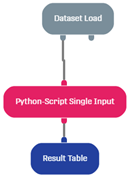Procedure:

• Dataset is split into Training (80 %) and Test Subset (20 %)
• Defining the Models to be used:
• Logistic Regression
• Linear Discriminant Analysis
• K Neighbors Classifier
• Decision Tree Classifier
• Gaussian Naive Bayes
• Support Vector Machine
• Evaluating each Model and printing the output
• Testing the Models on the validation set

Output:

• Lines 35-47 produce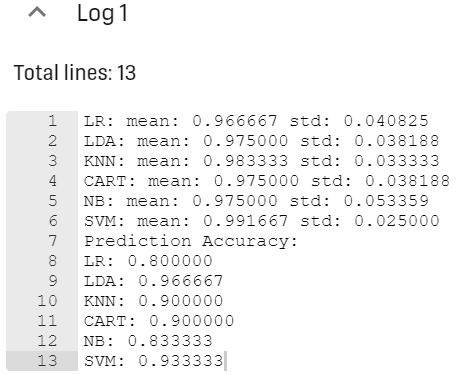## Using an Exposed Credential Key

An exposed Key's information (username, password, etc.) can be retrieved via API in Functions and Python Processors. So, if a Function requires Credentials to gain access to some service, it is possible to do this without writing them in plaintext. More on exposed Keys here

The following code snippet first retrieves then prints the Key's properties.

```# required package for sending requests
import requests
# create the header for the request using authorization
# performing a get request to retrieve the exposed key
# parse json result and read the keyInformation
if 'keyInformation' in r.json():
print(r.json()["keyInformation"])
else:
print(r.json()["errors"])```

Using the Python Script Data Generator Processor with the "Generate Empty Dataset Output" switch turned on and the above script, the following information gets logged inside the Processor.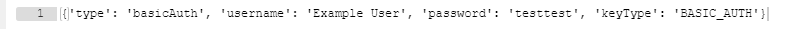# Related Articles

One Data Python Framework (ODPF)